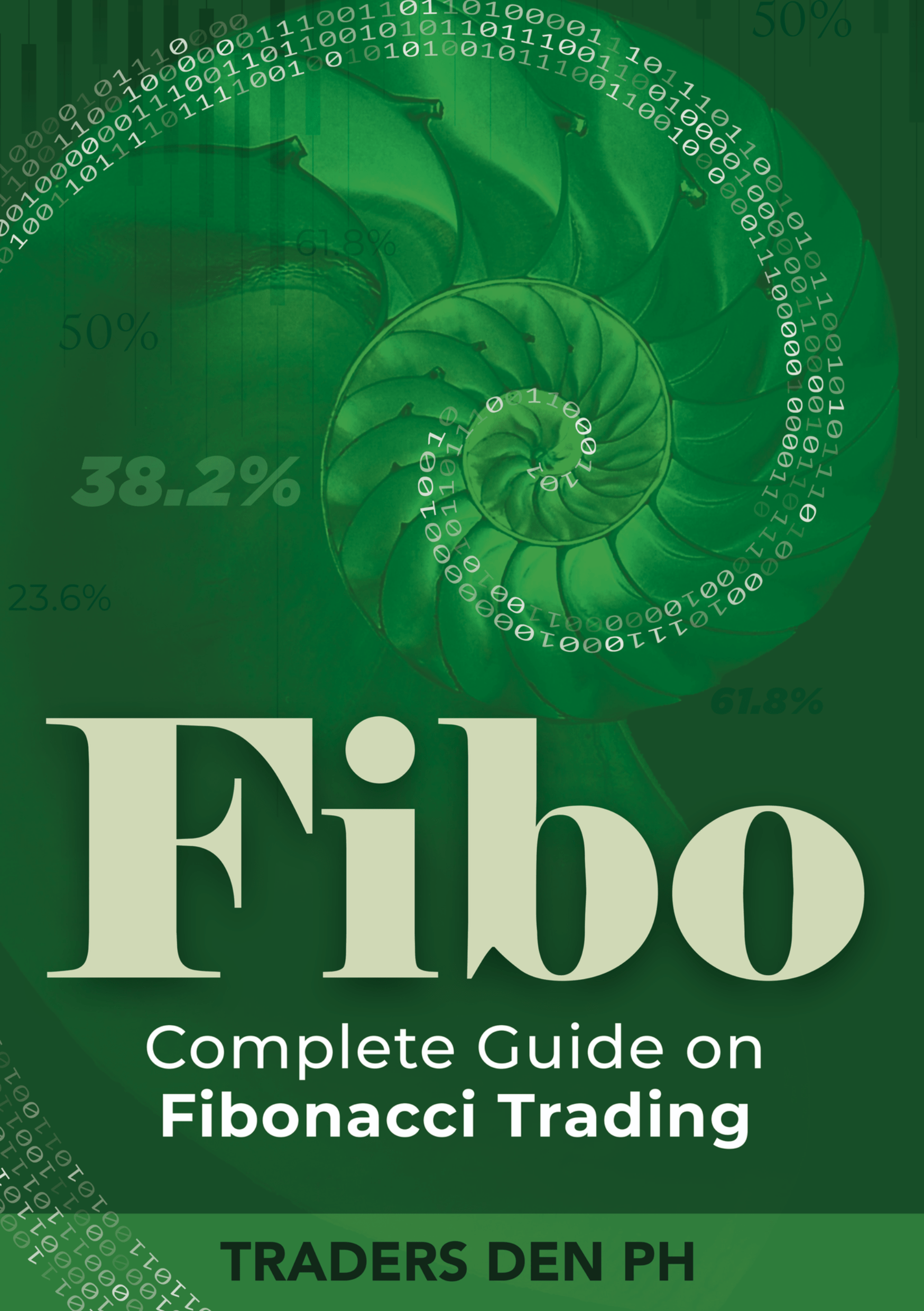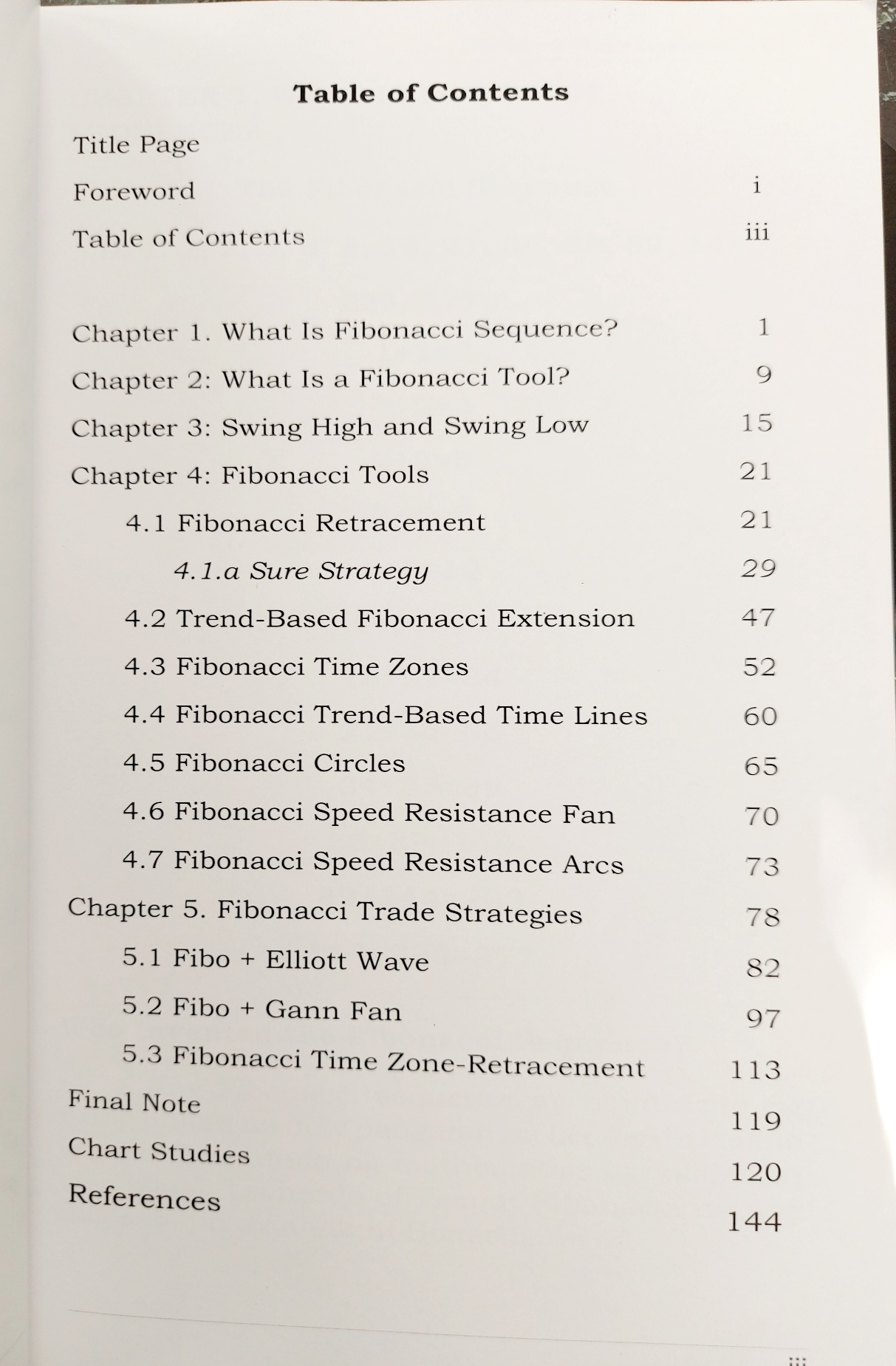# FIBO

Fibo is a complete book about Fibonacci Tool. Most traders only know Fibonacci Retracements. But there are a lot more Fibonacci Tools other than Fibonacci Retracement. This book will tell you everything there is to know about Fibonacci Tools.

FIBO is by far the most complete Fibonacci Trading book ever made available for Pinoy. After reading this book, you will surely  master the FIBONACCI tools.

# FIBO

Fibo is a complete book about Fibonacci Tool. Most traders only know Fibonacci Retracements. But there are a lot more Fibonacci Tools other than Fibonacci Retracement. This book will tell you everything there is to know about Fibonacci Tools.

FIBO is by far the most complete Fibonacci Trading book ever made available for Pinoy. After reading this book, you will surely  master the FIBONACCI tools.

#### Read the sneak peek below!###### Sneak Peek